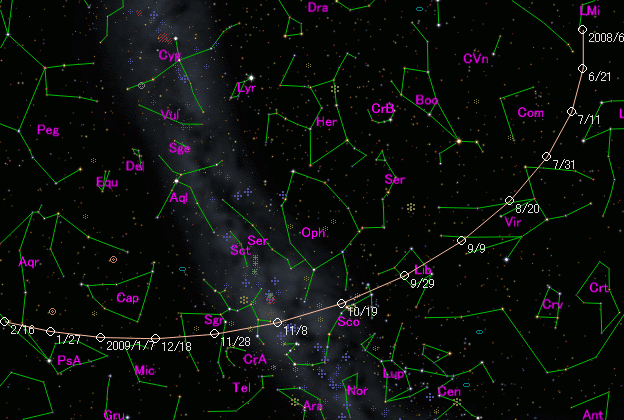# 7P/Pons-Winnecke (2008)###Pictures###Orbital Elements

```The following orbital elements are taken from MPC 54170:

Epoch 2008 Sept. 11.0 TT = JDT 2454720.5
T 2008 Sept. 26.6342 TT                                 MPC
q   1.253265             (2000.0)            P               Q
n   0.1549729      Peri.  172.3293      -0.0640958      +0.9231974
a   3.432671       Node    93.4229      -0.9345704      +0.0776315
e   0.634901       Incl.   22.3102      -0.3499569      -0.3764040
P   6.36
From 165 observations 1964-2004, mean residual 0".9.  Nongravitational
parameters A1 = +0.01, A2 = +0.0021.
```

###Finding Charts###Magnitudes Graph

```        H = 15.8  G = 0.15                         [    ,-400]  (             - 2007 Aug. 23)
m1 = 11.7 + 5 log d +  12.5 log r(t - 50)  [-400,-126]  (2007 Aug. 23 - 2008 May  23)
m1 = 55   + 5 log d - 150   log r          [-126,-115]  (2008 May  23 - 2008 June  3)
m1 = 11.7 + 5 log d +  12.5 log r(t - 50)  [-115, -80]  (2008 June  3 - 2008 July  8)
m1 =  5.5 + 5 log d +  50   log r          [ -80,  54]  (2008 July  8 - 2008 Nov. 19)
m1 = 11.5 + 5 log d +  15.0 log r(t - 50)  [  54, 380]  (2008 Nov. 19 - 2009 Oct. 11)
H = 15.8  G = 0.15                         [ 380,    ]  (2009 Oct. 11 -             )
```##### The orbital elements are published at the MPC Ephemerides and Orbital Elements. The charts are made with StellaNavigator Ver.8 (AstroArts). The magnitudes graphs are made with Comet for Windows.